login  home  contents  what's new  discussion  bug reports help  links  subscribe  changes  refresh  edit

 Submitted by : (unknown) at: 2007-11-17T21:55:49-08:00 (15 years ago) Name : Axiom Version : default friCAS-20090114 Axiom-20050901 OpenAxiom-20091012 OpenAxiom-20110220 OpenAxiom-Release-141 Category : Axiom Aldor Interface Axiom Compiler Axiom Library Axiom Interpreter Axiom Documentation Axiom User Interface building Axiom from source lisp system MathAction Doyen CD Reduce Axiom on Windows Axiom on Linux Severity : critical serious normal minor wishlist Status : open closed rejected not reproducible fix proposed fixed somewhere duplicate need more info Optional subject :   Optional comment :

Original Issue Report

axiom
digamma 2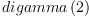(1)
Type: Expression(Integer)

Has to return digamma(2) (EXPR INT)

## Analysis

The statement of the issue is to terse and vague to be sure what the author had in mind, but assuming that s/he expected the result to be of type EXPR INT, here is how to see what is going on. Use the option )set message selection on to trace how Axiom finds the signature of the appropriate digamma function:

axiom
)set message selection on
digamma 2
Function Selection for digamma
Arguments: PI
  signature:   EXPR(INT) -> EXPR(INT)
implemented: slot $$from EXPR(INT)  signature: DFLOAT -> DFLOAT implemented: slot (DoubleFloat)(DoubleFloat) from DFSFUN  signature: COMPLEX(DFLOAT) -> COMPLEX(DFLOAT) implemented: slot (Complex (DoubleFloat))(Complex (DoubleFloat)) from DFSFUN(2) Type: Expression(Integer) Axiom finds 3 possible signatures and applies the first one because 2 can be coerced to DoubleFloat?. But we can ask Axiom to take the third option by either specifically treating the type of the input 2 as EXPR INT or by asking for something of type EXPR INT as the result. axiom digamma(2::EXPR INT) Function Selection for digamma Arguments: EXPR(INT)  signature: EXPR(INT) -> EXPR(INT) implemented: slot$$ from EXPR(INT)(3)
Type: Expression(Integer)
axiom
digamma(2)@(EXPR INT)
Function Selection for digamma
Arguments: EXPR(INT)
Target type: EXPR(INT)
-> no appropriate digamma found in Integer
  signature:   EXPR(INT) -> EXPR(INT)
implemented: slot $$from EXPR(INT)(4) Type: Expression(Integer) Of course as something of type EXPR INT this expression has no simplier representation. this works as documented --Bill Page, Wed, 23 Mar 2005 16:17:27 -0600 reply Status: open => rejected Sorry to respond to this but in Axiom, all trigonometric, transcendental etc.. functions is returned in EXPR INT if there is no integer functions. I think that Axiom has to return digamma(2) and digamma(2.0) the SF result. This permit to work directly on expression. You can test some other symbolic CAS.But may be I'm wrong. PLEASE RESPOND and THANK YOU --Bill Page, Wed, 23 Mar 2005 18:06:05 -0600 reply Please do not be "sorry to respond" - that is the purpose of this website! By the way, I think it would be polite (but it is not necessary) for you identify yourself by clicking on preferences rather than remaining anonymous. What I think you mean is this: Why is the mode selection for the following pairs of expressions different? axiom )set message selection on sin(2) Function Selection for sin Arguments: PI -> no appropriate sin found in PositiveInteger -> no appropriate sin found in Integer -> no appropriate sin found in PositiveInteger -> no appropriate sin found in Integer Modemaps from Associated Packages no modemaps Remaining General Modemaps  D -> D from D if D has TRIGCAT  signature: EXPR(INT) -> EXPR(INT) implemented: slot$$ from EXPR(INT)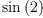(5)
Type: Expression(Integer)
axiom
digamma(2)
Function Selection for digamma
Arguments: PI
  signature:   EXPR(INT) -> EXPR(INT)
implemented: slot $$from EXPR(INT)  signature: DFLOAT -> DFLOAT implemented: slot (DoubleFloat)(DoubleFloat) from DFSFUN  signature: COMPLEX(DFLOAT) -> COMPLEX(DFLOAT) implemented: slot (Complex (DoubleFloat))(Complex (DoubleFloat)) from DFSFUN(6) Type: Expression(Integer) and axiom sin(2.0) Function Selection for float Arguments: (INT,INT,PI) Target type: FLOAT From: FLOAT  signature: (INT,INT,PI) -> FLOAT implemented: slot (Integer)(Integer)(PositiveInteger) from FLOAT Function Selection for sin Arguments: FLOAT  signature: FLOAT -> FLOAT implemented: slot$$ from FLOAT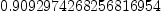(7)
Type: Float
axiom
digamma(2.0)
Function Selection for float
Arguments: (INT,INT,PI)
Target type: FLOAT
From:      FLOAT
  signature:   (INT,INT,PI) -> FLOAT
implemented: slot $(Integer)(Integer)(PositiveInteger) from FLOAT Function Selection for digamma Arguments: FLOAT -> no appropriate digamma found in Float -> no appropriate digamma found in Float Modemaps from Associated Packages  Complex(DoubleFloat) -> Complex(DoubleFloat) from DoubleFloatSpecialFunctions  DoubleFloat -> DoubleFloat from DoubleFloatSpecialFunctions  signature: DFLOAT -> DFLOAT implemented: slot (DoubleFloat)(DoubleFloat) from DFSFUN  signature: COMPLEX(DFLOAT) -> COMPLEX(DFLOAT) implemented: slot (Complex (DoubleFloat))(Complex (DoubleFloat)) from DFSFUN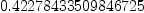(8) Type: DoubleFloat? Since sin is defined in DoubleFloat? and digamma is defined in DoubleFloatSpecialFunctions? one should expect similar treatment. axiom )show DoubleFloat DoubleFloat is a domain constructor Abbreviation for DoubleFloat is DFLOAT This constructor is exposed in this frame. ------------------------------- Operations -------------------------------- ?*? : (Fraction(Integer),%) -> % ?*? : (%,Fraction(Integer)) -> % ?*? : (%,%) -> % ?*? : (Integer,%) -> % ?*? : (PositiveInteger,%) -> % ?+? : (%,%) -> % ?-? : (%,%) -> % -? : % -> % ?/? : (%,Integer) -> % ?/? : (%,%) -> % ?<? : (%,%) -> Boolean ?<=? : (%,%) -> Boolean ?=? : (%,%) -> Boolean ?>? : (%,%) -> Boolean ?>=? : (%,%) -> Boolean Beta : (%,%) -> % D : % -> % D : (%,NonNegativeInteger) -> % Gamma : (%,%) -> % Gamma : % -> % OMwrite : (%,Boolean) -> String OMwrite : % -> String 1 : () -> % 0 : () -> % ?^? : (%,%) -> % ?^? : (%,Fraction(Integer)) -> % ?^? : (%,Integer) -> % ?^? : (%,PositiveInteger) -> % abs : % -> % acos : % -> % acosh : % -> % acot : % -> % acoth : % -> % acsc : % -> % acsch : % -> % airyAi : % -> % airyAiPrime : % -> % airyBi : % -> % airyBiPrime : % -> % angerJ : (%,%) -> % asec : % -> % asech : % -> % asin : % -> % asinh : % -> % associates? : (%,%) -> Boolean atan : (%,%) -> % atan : % -> % atanh : % -> % base : () -> PositiveInteger besselI : (%,%) -> % besselJ : (%,%) -> % besselK : (%,%) -> % besselY : (%,%) -> % bits : () -> PositiveInteger ceiling : % -> % coerce : Fraction(Integer) -> % coerce : Integer -> % coerce : Fraction(Integer) -> % coerce : % -> % coerce : Integer -> % coerce : % -> OutputForm convert : % -> InputForm convert : % -> Pattern(Float) convert : % -> DoubleFloat convert : % -> Float cos : % -> % cosh : % -> % cot : % -> % coth : % -> % csc : % -> % csch : % -> % differentiate : % -> % digamma : % -> % digits : () -> PositiveInteger ellipticE : (%,%) -> % ellipticE : % -> % ellipticF : (%,%) -> % ellipticK : % -> % ellipticPi : (%,%,%) -> % exp : % -> % exp1 : () -> % exponent : % -> Integer factor : % -> Factored(%) float : (Integer,Integer) -> % floor : % -> % fractionPart : % -> % gcd : List(%) -> % gcd : (%,%) -> % hankelH1 : (%,%) -> % hankelH2 : (%,%) -> % hash : % -> Integer hash : % -> SingleInteger inv : % -> % jacobiCn : (%,%) -> % jacobiDn : (%,%) -> % jacobiSn : (%,%) -> % jacobiTheta : (%,%) -> % kelvinBei : (%,%) -> % kelvinBer : (%,%) -> % kelvinKei : (%,%) -> % kelvinKer : (%,%) -> % kummerM : (%,%,%) -> % kummerU : (%,%,%) -> % lambertW : % -> % latex : % -> String lcm : List(%) -> % lcm : (%,%) -> % legendreP : (%,%,%) -> % legendreQ : (%,%,%) -> % lerchPhi : (%,%,%) -> % log : % -> % log10 : % -> % log2 : % -> % lommelS1 : (%,%,%) -> % lommelS2 : (%,%,%) -> % mantissa : % -> Integer max : (%,%) -> % min : (%,%) -> % negative? : % -> Boolean norm : % -> % nthRoot : (%,Integer) -> % one? : % -> Boolean order : % -> Integer pi : () -> % polygamma : (%,%) -> % polylog : (%,%) -> % positive? : % -> Boolean precision : () -> PositiveInteger prime? : % -> Boolean ?quo? : (%,%) -> % recip : % -> Union(%,"failed") ?rem? : (%,%) -> % retract : % -> Fraction(Integer) retract : % -> Integer riemannZeta : % -> % round : % -> % sample : () -> % sec : % -> % sech : % -> % sign : % -> Integer sin : % -> % sinh : % -> % sizeLess? : (%,%) -> Boolean smaller? : (%,%) -> Boolean sqrt : % -> % squareFree : % -> Factored(%) squareFreePart : % -> % struveH : (%,%) -> % struveL : (%,%) -> % tan : % -> % tanh : % -> % truncate : % -> % unit? : % -> Boolean unitCanonical : % -> % weberE : (%,%) -> % weierstrassP : (%,%,%) -> % weierstrassZeta : (%,%,%) -> % whittakerM : (%,%,%) -> % whittakerW : (%,%,%) -> % wholePart : % -> Integer zero? : % -> Boolean ?~=? : (%,%) -> Boolean ?*? : (NonNegativeInteger,%) -> % OMwrite : (OpenMathDevice,%,Boolean) -> Void OMwrite : (OpenMathDevice,%) -> Void ?^? : (%,NonNegativeInteger) -> % bits : PositiveInteger -> PositiveInteger if$ has ATARBPR
characteristic : () -> NonNegativeInteger
decreasePrecision : Integer -> PositiveInteger if $has ATARBPR differentiate : (%,NonNegativeInteger) -> % digits : PositiveInteger -> PositiveInteger if$ has ATARBPR
divide : (%,%) -> Record(quotient: %,remainder: %)
doubleFloatFormat : String -> String
euclideanSize : % -> NonNegativeInteger
expressIdealMember : (List(%),%) -> Union(List(%),"failed")
exquo : (%,%) -> Union(%,"failed")
extendedEuclidean : (%,%,%) -> Union(Record(coef1: %,coef2: %),"failed")
extendedEuclidean : (%,%) -> Record(coef1: %,coef2: %,generator: %)
float : (Integer,Integer,PositiveInteger) -> %
gcdPolynomial : (SparseUnivariatePolynomial(%),SparseUnivariatePolynomial(%)) -> SparseUnivariatePolynomial(%)
hypergeometricF : (List(%),List(%),%) -> % if $has RETRACT(INT) increasePrecision : Integer -> PositiveInteger if$ has ATARBPR
max : () -> % if not(has($,arbitraryExponent)) and not(has($,arbitraryPrecision))
meijerG : (List(%),List(%),List(%),List(%),%) -> % if $has RETRACT(INT) min : () -> % if not(has($,arbitraryExponent)) and not(has($,arbitraryPrecision)) multiEuclidean : (List(%),%) -> Union(List(%),"failed") patternMatch : (%,Pattern(Float),PatternMatchResult(Float,%)) -> PatternMatchResult(Float,%) precision : PositiveInteger -> PositiveInteger if$ has ATARBPR
principalIdeal : List(%) -> Record(coef: List(%),generator: %)
rationalApproximation : (%,NonNegativeInteger,NonNegativeInteger) -> Fraction(Integer)
rationalApproximation : (%,NonNegativeInteger) -> Fraction(Integer)
retractIfCan : % -> Union(Fraction(Integer),"failed")
retractIfCan : % -> Union(Integer,"failed")
subtractIfCan : (%,%) -> Union(%,"failed")
unitNormal : % -> Record(unit: %,canonical: %,associate: %)
weierstrassPPrime : (%,%,%) -> %
weierstrassSigma : (%,%,%) -> %

axiom
)show DoubleFloatSpecialFunctions
DoubleFloatSpecialFunctions  is a package constructor
Abbreviation for DoubleFloatSpecialFunctions is DFSFUN
This constructor is exposed in this frame.
------------------------------- Operations --------------------------------
Gamma : DoubleFloat -> DoubleFloat
Beta : (DoubleFloat,DoubleFloat) -> DoubleFloat
Beta : (Complex(DoubleFloat),Complex(DoubleFloat)) -> Complex(DoubleFloat)
Gamma : Complex(DoubleFloat) -> Complex(DoubleFloat)
airyAi : Complex(DoubleFloat) -> Complex(DoubleFloat)
airyAi : DoubleFloat -> DoubleFloat
airyBi : DoubleFloat -> DoubleFloat
airyBi : Complex(DoubleFloat) -> Complex(DoubleFloat)
besselI : (DoubleFloat,DoubleFloat) -> DoubleFloat
besselI : (Complex(DoubleFloat),Complex(DoubleFloat)) -> Complex(DoubleFloat)
besselJ : (DoubleFloat,DoubleFloat) -> DoubleFloat
besselJ : (Complex(DoubleFloat),Complex(DoubleFloat)) -> Complex(DoubleFloat)
besselK : (DoubleFloat,DoubleFloat) -> DoubleFloat
besselK : (Complex(DoubleFloat),Complex(DoubleFloat)) -> Complex(DoubleFloat)
besselY : (DoubleFloat,DoubleFloat) -> DoubleFloat
besselY : (Complex(DoubleFloat),Complex(DoubleFloat)) -> Complex(DoubleFloat)
digamma : DoubleFloat -> DoubleFloat
digamma : Complex(DoubleFloat) -> Complex(DoubleFloat)
hypergeometric0F1 : (DoubleFloat,DoubleFloat) -> DoubleFloat
hypergeometric0F1 : (Complex(DoubleFloat),Complex(DoubleFloat)) -> Complex(DoubleFloat)
logGamma : DoubleFloat -> DoubleFloat
logGamma : Complex(DoubleFloat) -> Complex(DoubleFloat)
polygamma : (NonNegativeInteger,DoubleFloat) -> DoubleFloat
polygamma : (NonNegativeInteger,Complex(DoubleFloat)) -> Complex(DoubleFloat)

please excuse my premature change of status --Bill Page, Wed, 23 Mar 2005 18:10:37 -0600 reply
Status: rejected => open

Treating special functions like Expression Integer --Bill Page, Wed, 23 Mar 2005 20:06:31 -0600 reply
Apparently the difference has something to do with the fact that DoubleFloat? is a domain while DoubleFloatSpecialFunctions? is a package. It is possible to obtain some of the effectst that you want by dropping the DoubleFloatSpecialFunctions? from the list of exposed constructors.
axiom
)set expose drop constructor DoubleFloatSpecialFunctions
DoubleFloatSpecialFunctions is now explicitly hidden in frame
initial

Then these are treated the same

axiom
sin(2)
Function Selection for sin
Arguments: PI
-> no appropriate sin found in PositiveInteger
-> no appropriate sin found in Integer
-> no appropriate sin found in PositiveInteger
-> no appropriate sin found in Integer
Modemaps from Associated Packages
no modemaps
Remaining General Modemaps
 D -> D from D if D has TRIGCAT
  signature:   EXPR(INT) -> EXPR(INT)
implemented: slot $$from EXPR(INT)(9) Type: Expression(Integer) axiom digamma(2) Function Selection for digamma Arguments: PI -> no appropriate digamma found in PositiveInteger -> no appropriate digamma found in Integer -> no appropriate digamma found in PositiveInteger -> no appropriate digamma found in Integer Modemaps from Associated Packages no modemaps Remaining General Modemaps  D -> D from D if D has SPFCAT  signature: EXPR(INT) -> EXPR(INT) implemented: slot$$ from EXPR(INT)(10)
Type: Expression(Integer)

except now it is necessary to do a package call to evaluate it even for something that is a floating point value.

axiom
digamma(2.0)
Function Selection for float
Arguments: (INT,INT,PI)
Target type: FLOAT
From:      FLOAT
  signature:   (INT,INT,PI) -> FLOAT
implemented: slot $(Integer)(Integer)(PositiveInteger) from FLOAT Function Selection for digamma Arguments: FLOAT -> no appropriate digamma found in Float -> no appropriate digamma found in Float Modemaps from Associated Packages no modemaps Remaining General Modemaps  D -> D from D if D has SPFCAT  signature: EXPR(FLOAT) -> EXPR(FLOAT) implemented: slot$$from EXPR(FLOAT)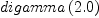(11) Type: Expression(Float) axiom digamma(2.0)$DoubleFloatSpecialFunctions
Function Selection for float
Arguments: (INT,INT,PI)
Target type: FLOAT
From:      FLOAT
  signature:   (INT,INT,PI) -> FLOAT
implemented: slot \$(Integer)(Integer)(PositiveInteger) from FLOAT
Function Selection for digamma
Arguments: FLOAT
From:      DFSFUN
  signature:   DFLOAT -> DFLOAT
implemented: slot (DoubleFloat)(DoubleFloat) from DFSFUN
  signature:   COMPLEX(DFLOAT) -> COMPLEX(DFLOAT)
implemented: slot (Complex (DoubleFloat))(Complex (DoubleFloat)) from DFSFUN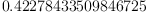(12)
Type: DoubleFloat?

Icomplete gamma function is missing --unknown, Thu, 24 Mar 2005 11:16:59 -0600 reply
In DoubleFloatSpecialFunctions?, incomplete gamma function is missing. If someone implements it, it's possible to add SpecialFunctionCategory? to the "Exports" of DoubleFloat? (add all these functions... => I don't know why gamma is actually exported by DoubleFloat?), unexpose DoubleFloatSpecialFunctions? and may be SpecialFunction?(Integer) will work as requested. The actual behavior is really annoying
Cheers

Category: Axiom Library => building Axiom from source Severity: serious => normal Status: open => planned

Category: building Axiom from source => Axiom Library Severity: normal => serious Status: planned => open

Status: open => closed

 Subject:   Be Bold !! ( 14 subscribers )